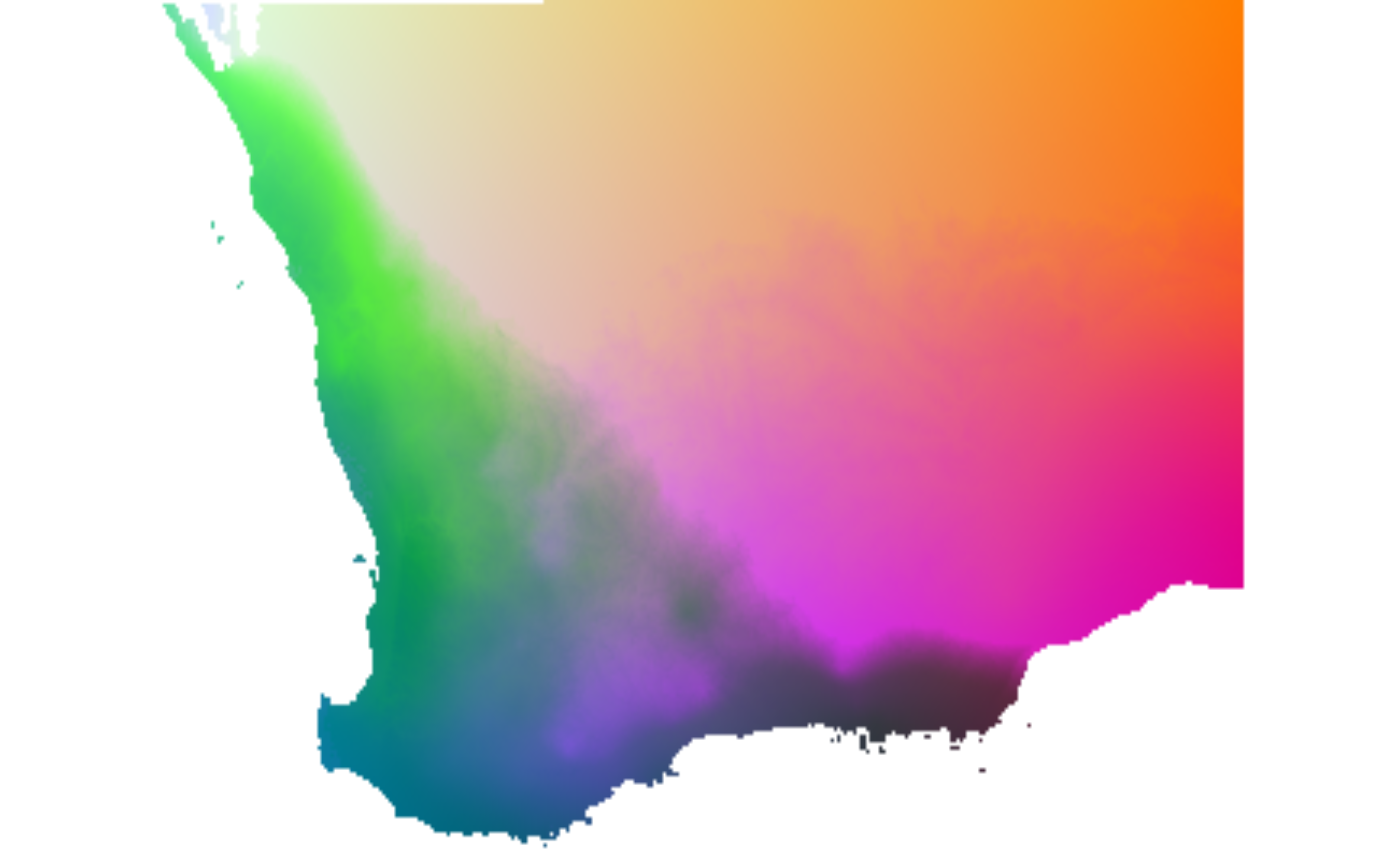This function transforms geographic and environmental predictors using (1) the fitted functions from a model object returned from gdm and (2) a data frame or raster stack containing predictor data for a set of sites.

gdm.transform(model, data)

## Arguments

model

A gdm model object resulting from a call to gdm.

data

Either (i) a data frame containing values for each predictor variable in the model, formatted as follows: X, Y, var1, var2, var3, ..., varN or (ii) a raster stack with one layer per predictor variable used in the model, excluding X and Y (rasters for x- and y-coordinates are built automatically from the input rasters if the model was fit with geo=T). The order of the columns (data frame) or raster layers (raster stack) MUST be the same as the order of the predictors in the site-pair table used in model fitting. There is currently no checking to ensure that the order of the variables to be transformed are the same as those in the site-pair table used in model fitting. If geographic distance was not used as a predictor in model fitting, the x- and y-columns need to be removed from the data to be transformed. Output is provided in the same format as the input data.

## Value

gdm.transform returns either a data frame with the same number of rows as the input data frame or a raster stack, depending on the format of the input data. If the model uses geographic distance as a predictor the output object will contain columns or layers for the transformed X and Y values for each site. The transformed environmental data will be in the remaining columns or layers.

## Examples

# start with the southwest data set
# grab the columns with xy, site ID, and species data
sppTab <- southwest[, c("species", "site", "Lat", "Long")]

##fit gdm using rasters
rastFile <- system.file("./extdata/swBioclims.grd", package="gdm")
envRast <- raster::stack(rastFile)
sitePairRast <- formatsitepair(sppTab, 2, XColumn="Long", YColumn="Lat", sppColumn="species",
siteColumn="site", predData=envRast)
#> Warning: No abundance column was specified, so the species data are
#>                 assumed to be presences.
#> Aggregation function missing: defaulting to length
#> Warning: When using rasters for prediction data, sites are assigned to the
#>               cells in which they are located and then aggreagted as necessary (e.g.,
#>               if more than one site falls in the same raster cell - common for rasters
#>               with large cells).
##remove NA values
sitePairRast <- na.omit(sitePairRast)

##fit raster GDM
gdmRastMod <- gdm(sitePairRast, geo=TRUE)

##raster input, raster output
transRasts <- gdm.transform(gdmRastMod, envRast)

# map biological patterns
rastDat <- raster::sampleRandom(transRasts, 10000)
pcaSamp <- prcomp(rastDat)

# note the use of the 'index' argument
pcaRast <- raster::predict(transRasts, pcaSamp, index=1:3)

# scale rasters
pcaRast[] <- (pcaRast[]-pcaRast[]@data@min) /
(pcaRast[]@data@max-pcaRast[]@data@min)*255
pcaRast[] <- (pcaRast[]-pcaRast[]@data@min) /
(pcaRast[]@data@max-pcaRast[]@data@min)*255
pcaRast[] <- (pcaRast[]-pcaRast[]@data@min) /
(pcaRast[]@data@max-pcaRast[]@data@min)*255

raster::plotRGB(pcaRast, r=1, g=2, b=3)